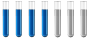## calculation of molar fraction and pressure

Chemistry and homework help forum.

Organic Chemistry, Analytical Chemistry, Biochemistry, Physical Chemistry, Computational Chemistry, Theoretical Chemistry, High School Chemistry, Colledge Chemistry and University Chemistry Forum.

Share your chemistry ideas, discuss chemical problems, ask for help with scientific chemistry questions, inspire others by your chemistry vision!

Please feel free to start a scientific chemistry discussion here!

Discuss chemistry homework problems with experts!

Ask for help with chemical questions and help others with your chemistry knowledge!

Moderators: expert, ChenBeier, Xen

anto007
Sr. MemberPosts: 25
Joined: Tue Aug 10, 2021 10:13 am
Location: Italy

### calculation of molar fraction and pressure

Hi everyone, i need your help again. I have this exercise: "In a closed reactor of 8.00 dm ^ 3, thermostated at a certain temperature, 2.50 dm ^ 3 of methane, CH4, measured at the pressure of 1.45 * 10 ^ 5 Pa, are mixed with 4.50 dm ^ 3 of oxygen, measured at 2.70 * 10 ^ 5 Pa and at the same temperature. A spark ignites the combustion of methane:
CH4 (g) + 2O2 (g) -> CO2 (g) + 2H2O (g)
calculate a) the total pressure and partial pressures of the gases before combustion; b) the molar fractions and partial pressures after combustion, knowing that at this temperature the water is in the form of vapor. "

I solved point a) like this:
I apply Boyle's isothermal law P1V1=P2V2
P1(CH4)=1.45 * 10 ^ 5 Pa
V1(CH4)=2.50 dm^3
V2= 8.00 dm^3
P2(CH4)=(P1(CH4)*V1(CH4))/V2=(1.45 * 10 ^ 5 Pa*2.50 dm^3)/8.00 dm^3= 4.53*10^4 Pa

P1(O2)=2.70 * 10 ^ 5 Pa
V1(O2)= 4.50 dm^3
V2= 8.00 dm^3
P2(O2)=(P1(O2)*V1(O2))/V2= (2.70 * 10 ^ 5 Pa* 4.50 dm^3)/8.00dm^3= 1.52*10^5 Pa

P(tot)= P2(CH4)+P2(O2)=(4.53*10^4 Pa)+(1.52*10^5 Pa)= 1.973*10^5 Pa

b)
Now to calculate the molar fractions I need to know the total moles and the moles of the individual gases of the products, but I can't figure out how to get them from the information I have. I know the water vapor temperature is 100 ° C (373.15 F). Could you help me understand how to extrapolate this information? Thank you very much
The mind is like a parachute, it only works if it opens. -A. Einstein
ChenBeier
Distinguished MemberPosts: 873
Joined: Wed Sep 27, 2017 7:25 am
Location: Berlin, Germany

### Re: calculation of molar fraction and pressure

Consider the vessel is 8 dm^3. In this is filled 2,5 dm^3 CH4 and 4.5 dm^3 O2 . In total 7 dm^3. 1 dm^3 is missing so we have some expansion.
of the gases. The two pressures are also different.

p1V1 + p2V2 = ptotal* Vvessel

pV ~ n. RT is constant.

From this you have to get total pressure and the partial pressures.
After burning you get new products, but what is really different , compare the moles.
anto007
Sr. MemberPosts: 25
Joined: Tue Aug 10, 2021 10:13 am
Location: Italy

### Re: calculation of molar fraction and pressure

Hello, sorry if I answer you late, but there was a fire emergency, here the woods have been burning for days.
Here is my development:
P(CH4)=1.45*10^5 Pa = 1.43 atm n(CH4)= (1.43 atm*2.50 L)/(0.08206*T) = 43.6/T mol
P(O2)= 2.7.*10^5 Pa = 2.66 atm n(O2) = (2.66 atm*4.5 L)/(0.08206*T) = 146.1/T mol
n tot = 189.6 mol
CH4 (g) + 2O2 (g) -> CO2 (g) + 2H2O (g)
43.6/T 146.1/T 0 0
-43.6/T -87.2/T +43.6/T +87.2/T
0 58.9/T 43.6/T 87.2/T
n tot =189.6/T
X(O2) = (58.9/T)/(189.6/T) = 0.311
X(CO2) = (43.6/T)/(189.6/T) = 0.230
X(H2O) = (87.2/T)/(189.6/T) = 0.460

Ptot= (189.6*0.08206*T)/(T*8) = 1.95 atm = 1.97*10^5 Pa
P(O2) = 0.311*1.97*10^5 Pa = 6.13*10^4 Pa
P(CO2) = 0.230*1.97*10^5 Pa = 4.53*10^4 Pa
P(H2O) = 0.460*1.97*10^5 Pa = 9.06*10^4 Pa
The mind is like a parachute, it only works if it opens. -A. Einstein
ChenBeier
Distinguished MemberPosts: 873
Joined: Wed Sep 27, 2017 7:25 am
Location: Berlin, Germany

### Re: calculation of molar fraction and pressure

I am really sorry to hear that a fire is close to your place. This planet is dying.